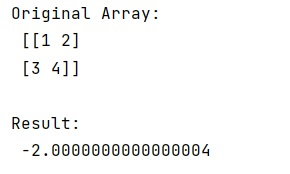# How to get the determinant of a matrix using NumPy?

Learn, how to calculate/get the determinant of a matrix using NumPy in Python?
Submitted by Pranit Sharma, on February 26, 2023

NumPy is an abbreviated form of Numerical Python. It is used for different types of scientific operations in python. Numpy is a vast library in python which is used for almost every kind of scientific or mathematical operation. It is itself an array which is a collection of various methods and functions for processing the arrays.

## Calculating the determinant of a matrix using NumPy

Mathematically, the determinant is a scalar value that is a function of the entries of a square matrix. It characterizes some properties of the matrix and the linear map represented by the matrix.

Suppose that we are given a numpy matrix and we need to find its determinant using numpy methods. Although there is no direct method to calculate the determinant of a matrix we can find out the determinant of a matrix in numpy using the numpy.linalg.det() method.

The linalg is a module in numpy where linalg stands for "linear algebra".

Let us understand with the help of an example,

## Python code to calculate the determinant of a matrix using NumPy

```# Import numpy
import numpy as np

# Creating a numpy array
arr = np.array([[1, 2], [3, 4]])

# Display original array
print("Original Array:\n",arr,"\n")

# Calculating determinant of the matrix
res = np.linalg.det(arr)

# Display result
print("Result:\n",res)
```

Output:Languages: » C » C++ » C++ STL » Java » Data Structure » C#.Net » Android » Kotlin » SQL
Web Technologies: » PHP » Python » JavaScript » CSS » Ajax » Node.js » Web programming/HTML
Solved programs: » C » C++ » DS » Java » C#
Aptitude que. & ans.: » C » C++ » Java » DBMS
Interview que. & ans.: » C » Embedded C » Java » SEO » HR
CS Subjects: » CS Basics » O.S. » Networks » DBMS » Embedded Systems » Cloud Computing
» Machine learning » CS Organizations » Linux » DOS
More: » Articles » Puzzles » News/Updates